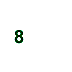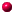Statistics: By the NumbersYou've seen the statistics...
 "9 out of 10 doctors recommend brand X" Contents of Statistics PagesNumbersCollection and AnalysisHow to Lie and Cheat "7 out of 10 people prefer the taste of brand Y" "The average person has an average number of Z every year"

 What do these numbers mean? Can you be mislead by statistics? The word "statistics" actually has several meanings. It can refer to single facts such as the number of people who like milk or the percentage of cats that is white. Statistics are used to describe groups of numbers. The methods and techniques used to collect, analyze and present a set of numbers are also called statistics...you may have heard people call this "number crunching." In research, statistics may be used to determine if a new drug or treatment is useful. In business, statistics may be used to make new products or services or to chart trends in public opinion. Let's take a closer look at "numbers" to see how they are collected, analyzed and displayed. If you know more about statistics, you should be able to make better decisions about what and whom to believe.Number Crunching?
But first, let's get two things straight:

1. You do not have to be a math expert to understand the basic concepts of statistics. A basic knowledge of math and a lot of common sense will be fine.

2. It's about the word "data." The word "data" is plural. You should say and write, "The data are ..." Do NOT say, "The data is..." If you are talking about just one number, the word is "datum."

## NumbersData that are actually collected are sometimes called "raw data." These are the numbers that have been measured and recorded. Suppose we wanted to find out if playing background music improves the running speed of rats in a maze. In the experiment, 11 rats (rat #1 - rat #11) would run while listening to music and 11 rats (rat #12 - rat #22) would run without listening to music. We would measure the time it takes each rat to run through the maze. The time (in seconds) for each rat to complete the maze is recorded.

Here are the raw data:

 MAZE RUNNING TIMES in seconds Music Group No Music Group Rat 1 = 11.1 Rat 2 = 18.3 Rat 3 = 18.2 Rat 4 = 22.8 Rat 5 = 11.4 Rat 6 = 33.3 Rat 7 = 18.8 Rat 8 = 26.3 Rat 9 = 29.7 Rat 10 = 28.5 Rat 11 = 30.9 Rat 12 = 23.2 Rat 13 = 22.6 Rat 14 = 10.3 Rat 15 = 15.7 Rat 16 = 11.9 Rat 17 = 9.9 Rat 18 = 11.1 Rat 19 = 29.3 Rat 20 = 34.2 Rat 21 = 23.6 Rat 22 = 11.0 There are several ways to describe and summarize these sets of numbers: the mean, median and mode.

Now let's crunch some numbers!

## The Mean

The "mean" is what we usually think of as an "average." The mean is simply the sum of all the scores in a group divided by the total number of scores. So, in our maze example:

The mean of the music group is

11.1 + 18.3 + 18.2 + 22.8 + 11.4 + 33.3 + 18.8 + 26.3 + 29.7 + 28.5 + 30.9=22.7
11

The mean of the "no music" group is

23.2 + 22.6 + 10.3 + 15.7 + 11.9 + 9.9 + 11.1 + 29.3 + 34.2 + 23.6 + 11.0=18.4
11

(Note that I have rounded off these numbers.)

## The Median

The median is another way to describe a set of numbers. The median is the score that is exactly midway in the set of numbers. The easiest way to find the median is to rank the numbers in order. If we rank the scores from the music group in the rat maze data, it would look like:

11.1, 11.4, 18.2, 18.3, 18.8, 22.8, 26.3, 28.5, 29.7, 30.9, 33.3

Therefore, the median (the midway score) is 22.8 because there are five scores higher than 22.8 (26.3, 28.5, 29.7, 30.9, 33.3) and five scores lower than 22.8 (11.4, 12.1, 18.2, 18.3, 18.8).

Why don't you determine the median of the "no music" group. Check your answer:

The median of the "no music" group is:

If you had an even number of scores in your data set (for example, the maze running times of 10 rats rather than 11 rats), the median would be the midway point between the two middle numbers. For example, in the set of numbers: 1, 2, 4, 6, 17, 20; the median is:

4 + 6=5
2When you have an odd number of scores, you don't even have to know how to add and divide to find the median. All you have to do is rank the numbers from low to high and find the middle number.

## Mode

The mode is a third way to describe a set of numbers. The mode is very easy to find; it is the number that occurs most often. For example in the set of numbers:

1, 4, 4, 4, 4, 4, 6, 6, 10, 11, 13, 15

the mode is 4 because it occurs the most times. The mode does not provide very much information about a whole set of numbers. It only tells what score occurs most frequently.

It is important to know the average of a group of numbers, but there is still more information to be squeezed out of the raw data. For example, it is important to know how similar a particular number is to the other numbers in the group. In other words, the amount of variation in the data can be determined. The two most common ways to describe variation are the range and the standard deviation.

## The Range

The range is the difference between the highest value and the lowest value in a sample. For example, in the set of numbers: 2, 2, 2, 7, 8, 9, 10, 11, 11, 15, 20, the range is:

20 - 2 = 18

Sometimes statisticians include the highest and lowest scores in the range. In this case, you must add "1" to the calculation. In other words:

20 - 2 + 1 = 19

The range is very easy to calculate, but it really does not give you very much information because it ignores most of the data. The range is only concerned with the highest and the lowest values.

## The Standard Deviation

The standard deviation is a very common method used in science to describe the variability in a set of numbers. It examines the spread (variability) of each data point around the mean. The standard deviation increases with an increase in the variability of the data. If every score in the data set are the same, then the standard deviation will equal zero.

 Continue to learn about statistics: how to collect data, how to analyze data, how to graph data and how to lie with statistics.Send E-mailGet NewsletterSearch Pages